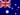All Modules View all topics Close topics
Fator polinômios sobre a forma de machado ^ 2 + bx + c

# Fator polinomiais no formulário ArmaniExchange ^ 2 + BX + C

• Nota de Estudos
• Rever TópicosRobert H. 1 0 - Module: Factor polynomials on the form of ax^2 + bx +c - Topic: Factor polynomials on the form AX^2 + BX + C Is there 2 answers to the video question? Factorise 2x(squared) + 10x + 12 Answer in video - (2x + 6) (x + 2) Another answer?? - (2x + 4) (x + 3)??### Você recebeu uma nova notificação

Clique aqui para visualizar todos eles